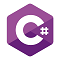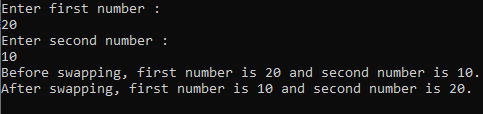# Snippets Zone#### Swap two numbers without using third variable in C#

850  28-June-2021

This snippet shows how to swap two numbers without using third variable in C#

In this snippet, we will see the program to swap two numbers without using the third variable in C#. Swapping program is one of the basic programs which is asked can be asked during exams or by the interviewer. In this snippet, we will swap two numbers by using simple mathematical calculations.

Program to swap two numbers without using the third variable:

``````static void Main(string[] args)
{
//Variable to hold an input number
int firstNumber;
int secondNumber;
Console.WriteLine("Enter first number :");
Console.WriteLine("Enter second number :");
//Before swapping
Console.WriteLine("Before swapping, first number is {0} and second number is {1}.", firstNumber, secondNumber);
//Swapping without using third variable
firstNumber = firstNumber + secondNumber;
secondNumber = firstNumber - secondNumber;
firstNumber = firstNumber - secondNumber;
//After swapping
Console.WriteLine("After swapping, first number is {0} and second number is {1}.", firstNumber, secondNumber);
//Console.ReadLine() to hold the screen after the execution of the program